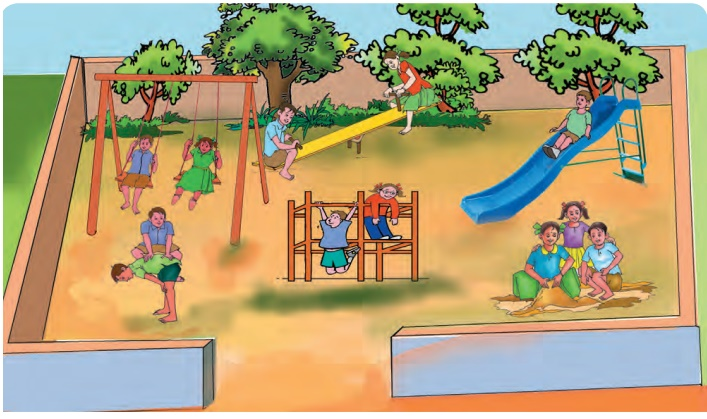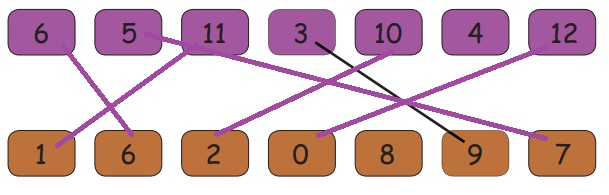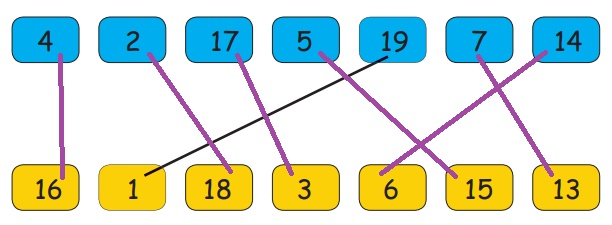Home | | Maths 1st Std | Addition

## Chapter: 1st Maths : Term 2 Unit 2 : Numbers

Teacher's Note: Teacher may ask more addition related questions based on the picture given above to recall the addition of numbers upto 9.

RecallNumber of children playing on the see-saw = 2

Number of children playing slide = 1

Total = 3

Teacher's Note: Teacher may ask more addition related questions based on the picture given above to recall the addition of numbers upto 9.5+1 =6

3+3=6

2+7=9

2+3 =5

1+6=7

4+5=9

Learn0+3=3

Know more

Zero added with any number gives the same number.

Practice1+0=1

0+2=2

0+3=3

4+0=4

5+0=5

0+6=6

7+0=7

0+8=8

9+0=9

Game

Teacher may arrange the paper cups on the table as shown in the picture. Give two chances for each student to hit the arranged cups from a particular distance using a ball. Note the number of cups hit by him/her in each chance. Finally ask them to count the total cups hit by them.Number of cups hit on the first chance = 4

Number of cups hit on the Second chance = 5

Number of cups hit in both the chances together = 9

Travel through

Keywords: Add, Total, Together, Altogether, More

Trees! How many trees?Mani and Kani are neighbours. They go to school together from their homes. One day on the way to school, Mani started counting the trees on his right side and said the total as 7. Kani also counted the trees on her left side and said the total as 5. When they reached the school, they told their friend Selvi about this.

Selvi told the number of trees that had been counted by Mani and Kani is 12. Kani and Mani were surprised and asked "How did you say that?"

Selvi’s way of countingTrees counted by Mani is 7     |  |  |  |  |  |  |  (Draw 7 lines)

Trees counted by Kani is 5     |  |  |  |  |  (Now draw 5 lines)

Total number of trees counted by both of them 12

|  |  |  |  |  |  |  |  |  |

| | (Total number of lines grouped in 10)

Learn

Using lines 6 + 9 = ? ; Using beads 12 + 4 = ?Practice1+9= 10

8+5= 13

15+0=15

7+7= 14

12+4= 11

19+0=19

14+3=17

11+7=18

6+ 7= 13

11+0=11

3+9= 12

16+4=20

4+13=17

17+1=18

Pleasure time

Find the total of the two numbers given in the table. Colour the peacock with  the colours indicated against the total.Think like a Mathematician

Match the pairs which make the sum 12?Match the pairs which make the sum 20?Mental math

→ Arasi has 9 pencils, Kamala has 8 pencils. How many pencils do they have altogether?

9+8=17

→ Mary plucked 7 mangoes, Agalya plucked 6 mangoes. They put the mangoes together in a basket. How many mangoes are there in the basket now?

7+6=13

→ Chezhiyan is 12 years old. His sister age is 3 years more than him. How old is Chezhiyan's sister?

12+3=15

→ Samirah has 8 crayons. She buys 4 more crayons. How many crayons does Samirah have totally?

8+4=12

Tags : Numbers | Term 2 Chapter 2 | 1st Maths , 1st Maths : Term 2 Unit 2 : Numbers
Study Material, Lecturing Notes, Assignment, Reference, Wiki description explanation, brief detail
1st Maths : Term 2 Unit 2 : Numbers : Addition | Numbers | Term 2 Chapter 2 | 1st Maths